# ISEE Middle Level Math : How to add fractions

## Example Questions

1 2 3 4 5 6 8 Next →

### Example Question #71 : How To Add Fractions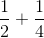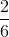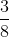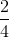Explanation:

When adding fractions, we must find a common denominator.  So, given the fractionswe see the denominators are 2 and 4.  The common denominator of 2 and 4 is 4.  So, we must make both denominators equal 4.  So,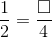How can we writeas a fraction with 4 in the denominator?  We must determine what number we can multiply the denominator by to get 4.  Once we determine that, we multiply the numerator by the same number.  So,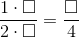So 2 times what number will give us 4?  The answer is 2.  So,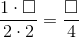Now that we know that, we must multiply the numerator by the same number.  So,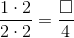and we get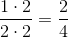Now, the 2 fractions have the same denominator, so we can add them.  So,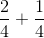When we add fractions, we add only the numerator and the denominator stays the same.  So,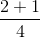### Example Question #72 : How To Add Fractions

Solve the following: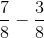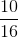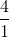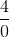Explanation:

To subtract fractions, we need to find a common denominator, then we subtract the numerators only.

So, given the problemwe can see we already have a common denominator. So, we will now subtract the numerators (note that we will leave the denominator alone). We get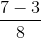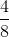### Example Question #73 : How To Add Fractions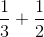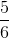Explanation:

To add fractions, we need to have a common denominator.  Then, we will add only the numerators, and we will leave the denominators alone.

So, given the problemwe will find a common denominator. To do that, we will find the least common multiply.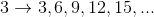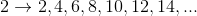We can see the least common multiply is 6. So, the denominator will be 6.

If we change the denominators to 6, we have to adjust the numerators accordingly. So, we get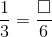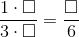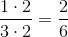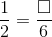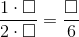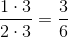Now, we can add. We get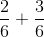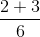### Example Question #74 : How To Add Fractions

Solve the following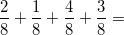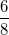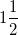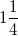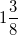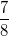Explanation:

To add fractions, we first add the numerators, and the denominator stays the same because it is the whole.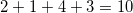.

This gives us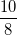.

This is equal to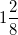which is reduced to### Example Question #75 : How To Add Fractions

Solve: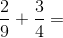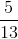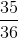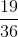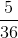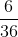Explanation:

Find the least common denominator betweenand. In this case, it is.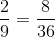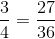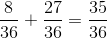1 2 3 4 5 6 8 Next →